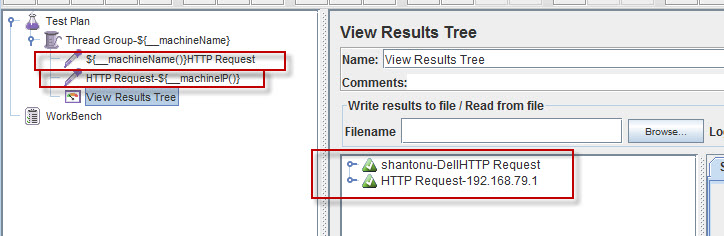In this article we are going to see functions of jmeter used for providing the PC information where jmeter test is running.

Why this is important? It is very important when
-we need to know where the test is runnning
-We need to identify specific request's environment
-We need to use the host information in the reports
-We need identify host to send or receive data.

Jmeter provides some build in set of functions that we can see. I will post a different post for basic idea on jmeter function and a function helper UI for that. In here here we sill see only necessary functions.

- To know the host's IP(where the jmeter is running)
\${__machineIP}
Note : \${__machineIP()} This will also work

- To know the host's Name (where the jmeter is running)
\${__machineName}
Note : \${__machineName()} This will also work

How can we use?
In place where we need to the function, it means, if we need to identity with a particular thread name, We will add this function before/after the thread name.
In case of any request(such as http), we will do the same. Or, we may use this in pre processor or post processor if we need.This is very useful while we are using Jmeter remotely with multiple hosts for running. We can get which request from which server using this.

Thanks..:)